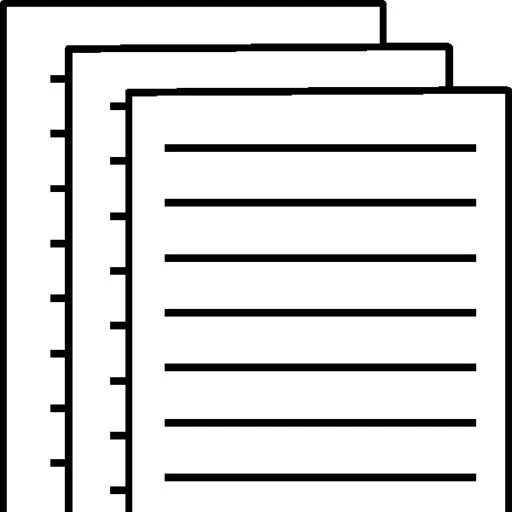## Finance

Finance

Name

Course

Instructor

Date

P6. Determine the present values (PVs) if \$5,000 is received in the future (i.e., at the end of each indicated time period) in each of the following situations:

P9. Assume you are planning to invest \$5,000 each year for six years and will earn 10 percent per year. Determine the future value (FV) of this annuity if your first \$5,000 is invested at the end of the first year.

1. 5000/(1+0.05)^10 = \$3070
2. 5000/(1+0.07)^7 = \$3114
3. 5000/1+0.09)^4 = \$3542

P10. Determine the present value (PV) now of an investment of \$3,000 made one year from now and an additional \$3,000 made two years from now if the annual discount rate is 4 percent.

1. FV=(5000((1.1)^6-1)/.1 = \$38578.05
2. P11. What is the present value (PV) of a loan that calls for the payment of \$500 per year for six years if the discount rate is 10 percent and the first payment will be made one year from now? How would your answer change if the \$500 per year occurred for ten years?

1. \$3,000/1.04+ \$3000/(1.04)^2=
2. \$2,884.62 + \$2,773.67 = \$5,658.29
3. P12. Determine the annual payment on a \$500,000, 12 percent business loan from a commercial bank that is to be amortized over a five-year period.

1. 10% for 6 years \$500.00 (4.355)= \$2,177.50
2. 10% for 10 years \$500.00 (6.145) = \$3,072.50
3. P13. Determine the annual payment on a \$15,000 loan that is to be amortized over a four-year period and carries a 10 percent interest rate. Prepare a loan amortization schedule for this loan.

1. 500,000{.12(1+.12)^5/(1+.12)^5-1} = 500,000{.12(1.12)^5/(1.12)^5-1} =
2. 500,000{.12(1.7623)/1.7623-1} = 500,000{.2114/.7623} = 500,000(.2774) = A = \$138,709.1696
3. Year PaymentPaymentRepaymentBalance

1. \$15,000/3.170 = \$4,732 (rounded to whole dollars)
2. AnnualInterestPrincipalLoan
3. 0\$0\$0\$0\$15,000

1\$4,732\$1,500\$3,232\$11,768

2\$4,732\$1,177\$3,555\$8,213

3\$4,732\$821\$3,911\$4,302

4\$4,732\$430\$4,302\$0

P15. Assume a bank loan requires an interest payment of \$85 per year and a principal payment of \$1,000 at the end of the loan’s eight-year life.

To view and download a complete answer, scroll down to the bottom to pay